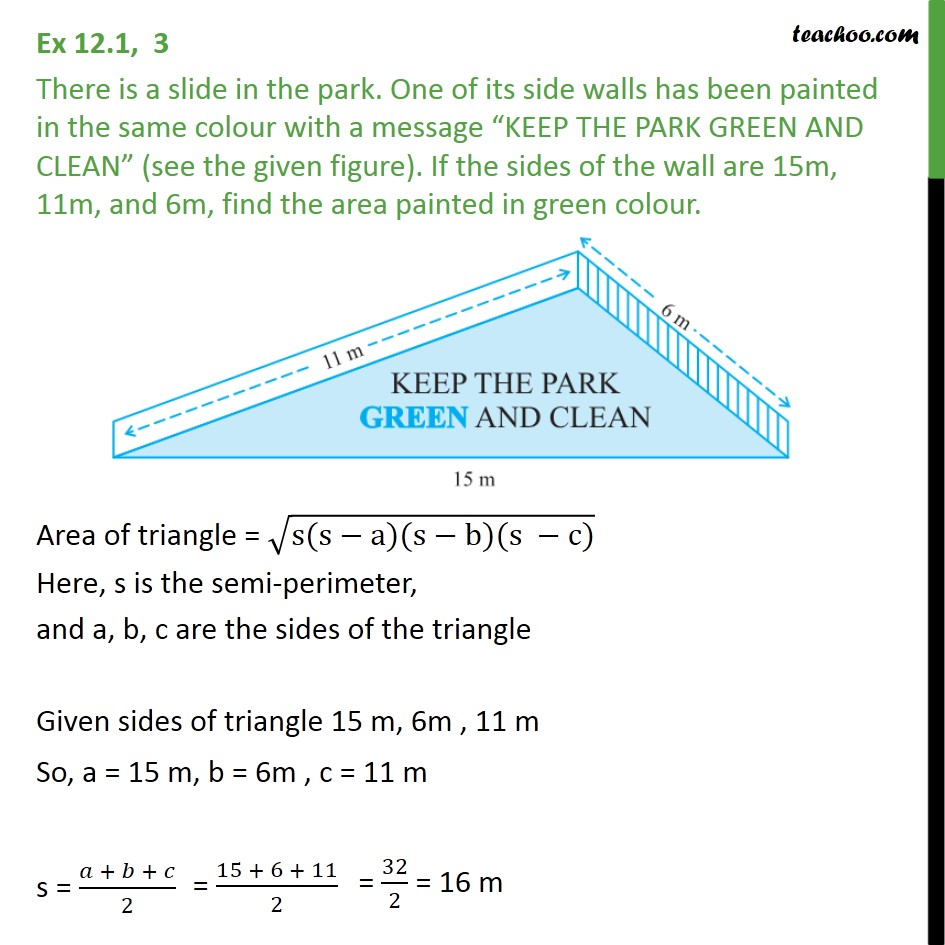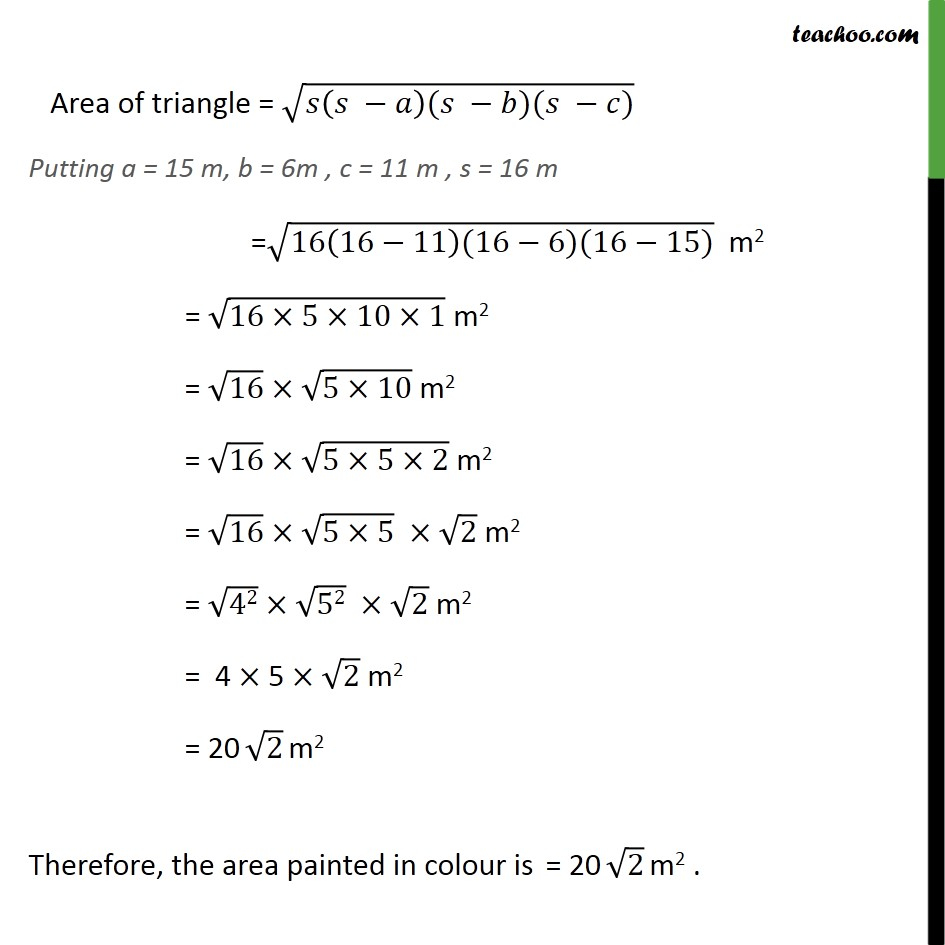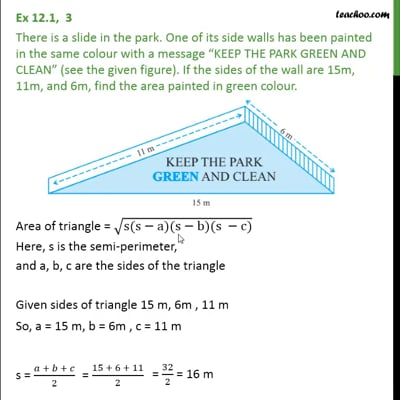Ex 12.1

Chapter 12 Class 9 Herons Formula
Serial order wiseThis video is only available for Teachoo black users

Solve all your doubts with Teachoo Black (new monthly pack available now!)

### Transcript

Ex 12.1, 3 There is a slide in the park. One of its side walls has been painted in the same colour with a message “KEEP THE PARK GREEN AND CLEAN” (see the given figure). If the sides of the wall are 15m, 11m, and 6m, find the area painted in green colour. Area of triangle = √(s(s−a)(s−b)(s −c)) Here, s is the semi-perimeter, and a, b, c are the sides of the triangle Given sides of triangle 15 m, 6m , 11 m So, a = 15 m, b = 6m , c = 11 m s = (𝑎 + 𝑏 + 𝑐)/2 Area of triangle = √(𝑠(𝑠 −𝑎)(𝑠 −𝑏)(𝑠 −𝑐)) Putting a = 15 m, b = 6m , c = 11 m , s = 16 m =√(16(16−11)(16−6)(16−15)) m2 = √(16×5×10×1) m2 = √16×√(5×10) m2 = √16×√(5×5×2) m2 = √16×√(5×5) " "×√2 m2 = √42×√52 " "×√2 m2 = 4 × 5 ×√2 m2 = 20 √2 m2 Therefore, the area painted in colour is = 20 √2 m2 .